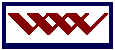WebWeevers.com CONTACT US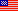DEO JUVANTE

 integrity flagstaff bmx flagstaff info faith terms of use All information provided within this website (complete) is offered as is and is not supported or specifically recommended over any other site. Should you have any questions, comments or otherwise, please click on this text, my picture above. to top

Fractals

Interactive fractals

The Fractal explorer below will zoom in on a given section chosen by YOU of the Mandelbrot Set.

The explorer will recalculate the given output for every new input and show you how Fractals continue forever into infinity.

What is a fractal?

A fractal is usually a rough or broken geometric shape which can be subdivided into parts.  A fractal is the result of the input and output of a formula in a special software program.

Fractals, described by Benoit Mandelbrot, is any image that is infinitely complex and self-similar at different levels. This is one definition of a fractal. A commonly used definition is something whose area is finite but whose perimeter is not.  Many fractals involve complex math.

Many computations of fractals involve complex numbers. Complex numbers are numbers with imaginary parts, which are in terms of "i" and a real number. "i" equals the square root of -1. Therefore, a complex number is often defined as a+b*i, where "a" and "b" are real numbers. To understand why this is necessary, try to compute the square root of negative one on a calculator, and see that it isn't possible with normal real numbers. To deal with this problem, mathematicians simply invented the quantity "i" to represent this. Complex numbers follow their own rules that sometimes differ from those of real numbers. Because of their unique properties, they are often used in fractals that are graphed in the complex plane, where the real number axis (a) lies horizontally and the imaginary axis (b) lies vertically. The Mandelbrot set is one example of a fractal that is graphed in the complex plane.

How to use the Mandelbrot Set Explorer below:

 INPUT:  Draw a rectangle within the Mandelbrot image (see example - right).  Then click "Go!"OUTPUT:  You'll get an amplification of the area inside the rectangle you created. Click the "col+" and "col-" buttons to change the color table.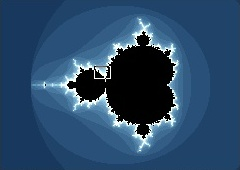The In and Out buttons let you zoom in and out through the explored regions. Explore the border between colored areas and the black set. The (Benoit) Mandelbrot Set Explorer

 NOTE:  This Mandelbrot explorer has an end because it is only for demonstration purposes.  This Mandelbrot Explorer does not even remotely come close to fully representing the power and vastness of what Fractals can do and really are in mathematics (geometry).This Fractal Explorer will not magnify once one value goes past E -15. History of fractals The Mandelbrot set--named after the man who accidentally discovered and coined the term "Fractals" in the early 1970's--is one of the most beautiful and profound discoveries in the history of mathematics.  Not since Pythagoras and the Greeks (circa 600 B.C.) who documented all their mathematical breakthroughs has there been such a revolutionary discovery. After his discovery, Benoit Mandelbrot used the Latin term "fractus," which means broken or irregular, to name fractals and called the new science the term "Fractals" because of the abstract fractional characteristics the formula produces. Fractals were not discovered until the invention of computers.  It was virtually impossible to discover fractals before the advent of the computer because of their complexity and gargantuan output. Fractals existed only in theory before the computer and were published in a most basic form in 1918 by Gaston Julia.  They were later published in an advanced form in 1925.  It is from this advanced formula that Benoit Mandelbrot made his now famous and revolutionary discovery. The following is from: Stephen Wolfram, A New Kind of Science Notes for Chapter 5: Two Dimensions and Beyond Section: Substitution Systems and Fractals Page 934 The idea of using nested 2D shapes in art probably goes back to antiquity; some examples were shown on page 43. In mathematics, nested shapes began to be used at the end of the 1800s, mainly as counterexamples to ideas about continuity that had grown out of work on calculus. The first examples were graphs of functions: the curve on page 920 was discussed by Bernhard Riemann in 1861 and by Karl Weierstrass in 1872. Later came geometrical figures: example (c) on page 191 was introduced by Helge von Koch in 1906, the example on page 187 by Waclaw Sierpinski in 1916, examples (a) and (c) on page 188 by Karl Menger in 1926 and the example on page 190 by Paul Lévy in 1937. Similar figures were also produced independently in the 1960s in the course of early experiments with computer graphics, primarily at MIT. From the point of view of mathematics, however, nested shapes tended to be viewed as rare and pathological examples, of no general significance. But the crucial idea that was developed by Benoit Mandelbrot in the late 1960s and early 1970s was that in fact nested shapes can be identified in a great many natural systems and in several branches of mathematics. Using early raster-based computer display technology, Mandelbrot was able to produce striking pictures of what he called fractals. And following the publication of Mandelbrot’s 1975 book, interest in fractals increased rapidly. Quantitative comparisons of pure power laws implied by the simplest fractals with observations of natural systems have had somewhat mixed success, leading to the introduction of multifractals with more parameters, but Mandelbrot’s general idea of the importance of fractals is now well established in both science and mathematics. The fractal universe Fractals, so far, appear to have no end and continue into true infinity.  There are, however, points on the geometric phenomena that boil down to zero (the black area).  All other areas that work toward infinity are represented in various colors depending on how fast they fade in or out of view. Fractals, in mathematics, are geometric shapes that are complex and detailed in structure at any level of magnification. Often fractals are self-similar—that is, they have the property that each small portion of the fractal can be viewed as a reduced-scale replica of the whole. One example of a fractal is the “snowflake” curve constructed by taking an equilateral triangle and repeatedly erecting smaller equilateral triangles on the middle third of the progressively smaller sides. Theoretically, the result would be a figure of finite area but with a perimeter of infinite length, consisting of an infinite number of vertices. In mathematical terms, such a curve cannot be differentiated (see Calculus). Many such self-repeating figures can be constructed, and since they first appeared in the 19th century they have been considered as merely bizarre. Fractal geometry is not simply an abstract development. A coastline, if measured down to its least irregularity, would tend toward infinite length just as does the “snowflake” curve. Mandelbrot has suggested that mountains, clouds, aggregates, galaxy clusters, and other natural phenomena are similarly fractal in nature, and fractal geometry's application in the sciences has become a rapidly expanding field. Practical use of fractals today The beauty of fractals has made them a key element in computer graphics.  Fractals have also been used to compress still and video images on computers. In 1987, English-born mathematician Dr. Michael F. Barnsley discovered the Fractal Transform™ which automatically detects fractal codes in real-world images (digitized photographs). The discovery spawned fractal image compression, used in a variety of multimedia and other image-based computer applications. Mathematics and the fractal Fractals are found within a branch of mathematics (geometry) concerned with irregular patterns made of parts that are in some way similar to the whole, e.g., twigs and tree branches, a property called self-similarity or self-symmetry. Unlike conventional geometry , which is concerned with regular shapes and whole-number dimensions, such as lines (one-dimensional) and cones (three-dimensional), fractal geometry deals with shapes found in nature that have non-integer, or fractal, dimensions—line-like rivers with a fractal dimension of about 1.2618 and cone-like mountains with a fractal dimension between 2 and 3. Fractal geometry developed from Benoit Mandelbrot's study of complexity and chaos (see chaos theory). Julia sets Julia sets sit in the complex plane, where the horizontal axis represents real numbers, and the vertical axis represents imaginary numbers. Each Julia set (there are an infinite number) is determined by its constant value, c, which is a complex number.  Now imagine you take another complex number, z. You will run this number through the following iterative equation. The formula behind fractals The simple formula first used by Benoit Mandelbrot: Z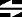Z2 + C (Z equals Z squared plus C) ...is the "recipe" for an extremely complicated output.  The formula used in the Mandelbrot Set is an iterative formula. That is to say, the meaning of iteration is: a procedure in which repetition of a sequence of operations yields results successively closer to a desired result. This "recipe" or formula will carry out a set of instructions until the parameters change, continue with secondary instructions until the parameters change again, and will do so until the sequence of operations is complete (including third, forth, fifth, sixth set of instructions, etc. if applicable). The discovery of fractals A turning point in the study of fractals came with the discovery of fractal geometry by the Polish-born French mathematician Benoit B. Mandelbrot in the 1970s. Mandelbrot adopted a much more abstract definition of dimension than that used in Euclidean geometry, stating that the dimension of a fractal must be used as an exponent when measuring its size. The result is that a fractal cannot be treated as existing strictly in one, two, or any other whole-number dimensions. Instead, it must be handled mathematically as though it has some fractional dimension. The “snowflake” curve of fractals has a dimension of 1.2618. Mandelbrot biography Benoit B. Mandelbrot - 1924-, French mathematician, born in Warsaw, Poland on November 20, 1924 to a Jewish family originally from Lithuania. He moved to France in 1936 and in 1944 entered college under Gaston Julia. Later, he worked at an IBM research center and studied chaotic data in economics. Largely self-taught and considered a maverick in the field of mathematics, he is uncomfortable with the rigorously pure logical analysis prescribed by Nicolas Bourbaki and relies instead on his talent for visualizing natural phenomena. A pioneer of chaos theory , he conceived, developed, and applied fractal geometry , which is used to find order in apparently erratic shapes and processes. Beginning in 1961, Benoit Mandelbrot published a series of studies on fluctuations of the stock market, the turbulent motion of fluids, the distribution of galaxies in the universe, and on irregular shorelines on the English coast. By 1975 Mandelbrot had developed a theory of fractals that became a serious subject for mathematical study. Fractal geometry has been applied to such diverse fields as the stock market, chemical industry, meteorology, and computer graphics .

 flagstaff photos iq tests hidalgo suicide photography tithing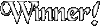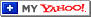notice © 1997+ All rights reserved Another webweevers.com Production A division of HidalCorp

 Quote of the Day #QuoteOfTheDay {width:100%;border:1px #999999 solid;background-color:#FFFFFF} #QuoteOfTheDay TD {font:normal 8pt '';color:#333366} #QuoteOfTheDay A {color:#FFFFFF} Quote of the Day provided by The Free Library This Day in History #TodaysHistory {width:100%;border:1px #999999 solid;background-color:#FFFFFF} #TodaysHistory H3 {margin-top:0px;font:bold 10pt '';color:#333366} #TodaysHistory TD {font:normal 8pt '';color:#333366} #TodaysHistory A {color:#FFFFFF} This Day in History provided by The Free Dictionary Hangman #Hangman {border:1px #999999 solid;background-color:#FFFFFF;height:240px} Hangman provided by The Free Dictionary

C. Czach Hidalgo 1996+ webweevers.com
"Fire @ F-Holes Cove"
Blue Ridge Reservoir, AZ
September 4, 2007